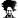# Where N > 2, Test to see if all N values are equal

#### polarisking

##### Member
Obvious answer uses the Transitive property we learned early on; you know, if A = B, and B= C then A = C. Could be written as

=AND(Cell01=Cell02, Cell02=Cell03) = True

For N values, though, is there an elegant using a CSE formula, perhaps? or something else?

#### Luke M

##### Excel Ninja
Perhaps something like
=COUNTIF(A:A, A1)=COUNT(A:A)

which checks if all the numbers in col A are equal to A1.

•polarisking

#### Peter Bartholomew

##### Well-Known Member
You could maintain the symmetry of the operation by using
= AND( range=TRANSPOSE(range) )
or, to avoid CSE, use a name 'identical' to hold the inner formula
{= ( range=TRANSPOSE(range) )}
and then
= AND( identical )
gives the result without CSE.
This works because it is only formulas on the grid that block array calculation by performing implicit intersection.

With modern dynamic arrays available within versions of Office 365, you could use UNIQUE and count the results
= ROWS( UNIQUE( range) ) = 1

p.s. If the cell contents are not numbers @Luke M's formula
= COUNTIF(range, cell01)=COUNTA(range)
would need COUNTA rather than COUNT.

#### NARAYANK991

##### Excel Ninja
Hi ,

Mathematically , if all the numbers in a set are the same , then SUM(all the numbers) = COUNT(all the numbers) * number.

=SUM(\$A\$1:\$A\$10) = COUNT(\$A\$1:\$A\$10)*A1

will be true if all the numbers are the same.

Narayan

•GraH - Guido

#### Luke M

##### Excel Ninja
Hi ,

Mathematically , if all the numbers in a set are the same , then SUM(all the numbers) = COUNT(all the numbers) * number.

=SUM(\$A\$1:\$A\$10) = COUNT(\$A\$1:\$A\$10)*A1

will be true if all the numbers are the same.

Narayan
But it's also true when the numbers are different. Consider {2, 3, 3, 4}.

•NARAYANK991

#### NARAYANK991

##### Excel Ninja
But it's also true when the numbers are different. Consider {2, 3, 3, 4}.
Hi Luke ,

I agree. Thanks for pointing this out.

Narayan

#### NARAYANK991

##### Excel Ninja
Hi ,

What about the minimum and the maximum in the set being equal ?

=MIN(\$A\$1:\$A\$10) = MAX(\$A\$1:\$A\$10)

Narayan

•polarisking

#### GraH - Guido

##### Well-Known Member
Since we are open to suggestions, why not use PQ?

Load table of data (numbers or not) to PQ and add an Index
Reference this first query and group by on the index "All Rows"
Add custom column "Transpose" with the following formula `= Table.FirstN(Table.Transpose([All]), Table.ColumnCount([All])-1)`
Add a second one "All Equal" (or wrap around first) `= List.Count(List.Distinct(Table.Column([Transpose], "Column1"))) = 1 `
Merge both tables on the Index and expand the "All Equal" column only

For reference; file attached.

Second alternative could be to remove "nulls" as well. List.Count(List.RemoveNulls(List.Distinct(Table.Column([Transpose], "Column1")))) = 1

Since PQ is case sensitive, the equal is also "exactly the same".

#### Attachments

• 19.5 KB Views: 0

#### polarisking

##### Member
I appreciate all these responses.

My specific situation is that I have 4 dates each contained in a variable name dimmed as DATE.

I suppose I could invoke a temporary step by writing them into a contiguous vertical or horizontal range, and do the COUNTIF technique. I was hopingthat someone has some obscure, super elegant way to do the N variable compare returning TRUE if all were equal, and false if any one weren't equal.
Hi ,

What about the minimum and the maximum in the set being equal ?

=MIN(\$A\$1:\$A\$10) = MAX(\$A\$1:\$A\$10)

Narayan
This is incredibly elegant. For numbers and dates, this works. Especially in VBA where the variables are discreet and not contiguous.

=worksheetfunction.min(val01,val02,val03,,,,valN) = worksheetfunction.max(val01,val02,val03,,,,valN)

Text values are a whole other animal . . .

#### Peter Bartholomew

##### Well-Known Member
This is still worksheet formula based rather than VBA. First multi-select every number you are interested in and then define the selection to be a named range 'multiCell'. @NARAYANK991's second solution (post #7) works fine with a multi-area range, so
= ( MIN(multiCell)=MAX(multiCell) )
gives the desired result.

#### Peter Bartholomew

##### Well-Known Member
It is no longer relevant but a formula to 'get all one's ducks in a row' is
= CHOOSE( {1,2,3}, cell01, cell02, cell03 )
The formula
= AND( array=TRANSPOSE(array) )
works on the resulting array.

•NARAYANK991